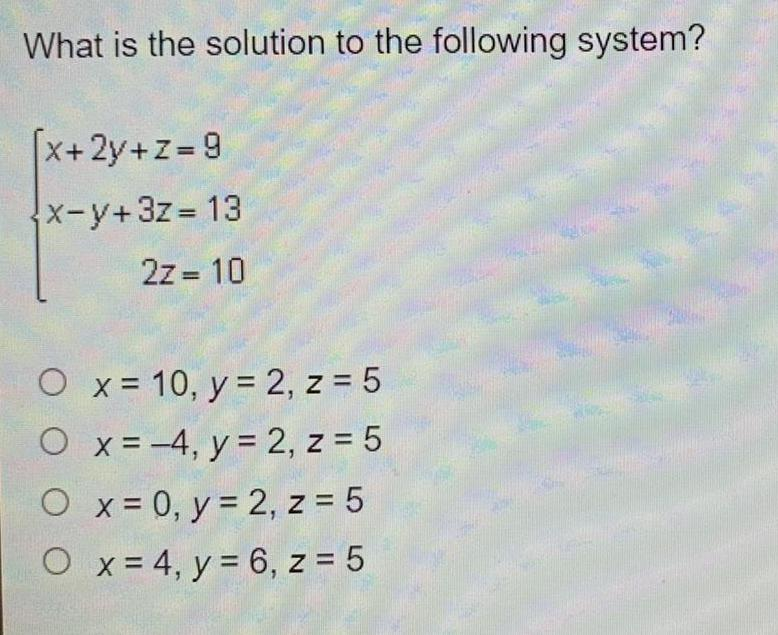Question:

# What is the solution to the following system? [x+2y+z= 9

Last updated: 7/4/2022What is the solution to the following system? [x+2y+z= 9 x-y+3z=13 2z = 10 x = 10, y = 2, z = 5 4, y = 2, z = 5 O x = 0, y = 2, z = 5 O x = 4, y = 6, z = 5 O O x = An